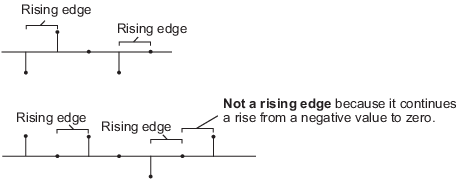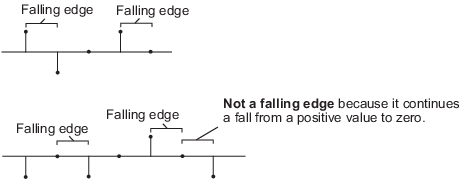# dsp.Delay

Delay input signal by fixed samples

## Description

The `dsp.Delay` System object™ delays the input by a specified number of samples along each channel (column) of the input. You can specify the initial output of the object through the InitialConditions property. To reset the delay, enable the ResetCondition through the ResetInputPort.

To delay the input:

1. Create the `dsp.Delay` object and set its properties.

2. Call the object with arguments, as if it were a function.

## Creation

### Syntax

``delay = dsp.Delay``
``delay = dsp.Delay(Name,Value)``
``delay = dsp.Delay(len,Name,Value)``

### Description

example

``delay = dsp.Delay` creates a System object that delays the input by 1 sample.`

example

````delay = dsp.Delay(Name,Value)` creates a delay System object with each specified property set to the specified value. Enclose each property name in single quotes.Example: ```delay = dsp.Delay('InitialConditionsPerChannel',true);``````

example

````delay = dsp.Delay(len,Name,Value)` creates a delay System object, `delay`, with the `Length` property set to `len`, and other specified properties set to the specified values. Enclose each property name in single quotes.Example: ```delay = dsp.Delay(10,'ResetInputPort',true,'ResetCondition','Rising edge');``````

## Properties

expand all

Unless otherwise indicated, properties are nontunable, which means you cannot change their values after calling the object. Objects lock when you call them, and the `release` function unlocks them.

If a property is tunable, you can change its value at any time.

Amount of delay in samples to apply to the input signal, specified as one of the following:

• Scalar positive integer –– The object applies equal delay to all the channels.

• Vector of positive integers –– The length of the vector must equal the number of input channels (columns). The object delays each channel by the amount specified by the respective element in the delay vector.

Enable different initial conditions per channel, specified as either:

• `false` –– The object applies the same initial conditions for all channels.

• `true` –– The object applies different initial conditions for each channel.

The value of this property must be the same as the value you choose for the `InitialConditionsPerSample` property. This value determines the size of the initial conditions array. For more details, see the InitialConditions property.

Different initial conditions per sample, specified as either:

• `false` –– The object applies the same initial conditions for all samples.

• `true` –– The object applies different initial conditions for each sample.

The value of this property must be the same as the value you choose for the `InitialConditionsPerChannel` property. This value determines the size of the initial conditions array. For more details, see the InitialConditions property.

Initial output of System object, specified as a scalar, vector, or a matrix. The dimensions of the initial condition matrix must be (`Length` property value)-by-(number of input channels).

If the input is an M-by-N matrix, the dimensions of the `InitialConditions` property value must be as follows:

`InputConditionsPerChannel``InputConditionsPerSample``InitialConditions`
`false``false`scalar
`true``true``Length`-by-N matrix

Enable the reset condition so that you can pass the reset control input to the object, specified as either:

• `false` –– The object does not reset the delay states.

• `true` –– You must pass a reset control input to the object by using the ResetCondition property. The object resets the delay states based on the values of the `ResetCondition` property and the reset control that is input to the object.

Event that triggers the reset of the delay, specified as one of the following. The object resets the delay whenever a reset event is detected in its reset input.

• `'Non-zero'` –– Triggers a reset operation at each sample, when the reset input is not zero.

• `'Rising edge'` –– Triggers a reset operation when the reset input does one of the following:

• Rises from a negative value to either a positive value or zero.

• Rises from zero to a positive value, where the rise is not a continuation of a rise from a negative value to zero.• `'Falling edge'` –– Triggers a reset operation when the reset input does one of the following:

• Falls from a positive value to a negative value or zero.

• Falls from zero to a negative value, where the fall is not a continuation of a fall from a positive value to zero.• `'Either edge'` –– Triggers a reset operation when the reset input is a rising edge or a falling edge.

#### Dependencies

This property applies only when you set the `ResetInputPort` property to `true`.

## Usage

### Syntax

``delayOut = delay(dataInput)``
``delayOut = delay(dataInput,resetInput)``

### Description

example

````delayOut = delay(dataInput)` adds delay to the data input and returns the delayed output. Each column of the input is treated as an independent channel.```

example

````delayOut = delay(dataInput,resetInput)` adds delay to the data input and selectively resets the state of the System object based on the value of the reset input and the value of the ResetCondition property.To pass the reset input, set ResetInputPort property to `true`.delay = dsp.Delay('ResetInputPort',true); ... delayOut = delay(dataInput,resetInput);```

### Input Arguments

expand all

Data input that is delayed by the object, specified as a vector or a matrix.

Example: [1;2;3;4;5]

Data Types: `single` | `double` | `int8` | `int16` | `int32` | `int64` | `uint8` | `uint16` | `uint32` | `uint64` | `logical` | `fi`
Complex Number Support: Yes

Reset input, specified as a scalar.

Example: 2

Data Types: `single` | `double` | `int8` | `int16` | `int32` | `uint8` | `uint16` | `uint32` | `logical`

### Output Arguments

expand all

Delayed output, returned as a vector or matrix. The size and data type of the output match the size and data type of the data input.

Example: [0;0;1;2;3]

Data Types: `single` | `double` | `int8` | `int16` | `int32` | `int64` | `uint8` | `uint16` | `uint32` | `uint64` | `logical` | `fi`
Complex Number Support: Yes

## Object Functions

To use an object function, specify the System object as the first input argument. For example, to release system resources of a System object named `obj`, use this syntax:

`release(obj)`

expand all

 `step` Run System object algorithm `release` Release resources and allow changes to System object property values and input characteristics `reset` Reset internal states of System object

## Examples

collapse all

Delay input by five samples by using the `dsp.Delay` System object™. By default, the initial conditions are 0.

Note: If you are using R2016a or earlier, replace each call to the object with the equivalent step syntax. For example, obj(x) becomes step(obj,x).

Delay input by 4 samples.

```delay = dsp.Delay(4); input = [(1:10)' (11:20)']; delayOut = delay(input) %#ok```
```delayOut = 10×2 0 0 0 0 0 0 0 0 1 11 2 12 3 13 4 14 5 15 6 16 ```
`release(delay);`

Specify initial conditions for each channel and for each sample. The `InitialConditions` property must be a (Length)-by-(NumChannels) matrix.

```delay.InitialConditionsPerChannel = true; delay.InitialConditionsPerSample = true; delay.InitialConditions = [(0.1:0.1:0.4)' (0.5:0.1:0.8)']; delayOut = delay(input) %#ok```
```delayOut = 10×2 0.1000 0.5000 0.2000 0.6000 0.3000 0.7000 0.4000 0.8000 1.0000 11.0000 2.0000 12.0000 3.0000 13.0000 4.0000 14.0000 5.0000 15.0000 6.0000 16.0000 ```
`release(delay);`

Reset the delay by setting the reset event to `'Rising edge'`. In this mode, a reset event occurs when the reset input:

• Rises from a negative value to 0.

• Rises from a negative value to a positive value.

• Rises form 0 to a positive value, where the rise is not a continuation from a negative value to 0.

Pass an initial reset input of 0.

```delay.ResetInputPort = true; delay.ResetCondition = 'Rising edge'; delayOut = delay(input,0) %#ok```
```delayOut = 10×2 0.1000 0.5000 0.2000 0.6000 0.3000 0.7000 0.4000 0.8000 1.0000 11.0000 2.0000 12.0000 3.0000 13.0000 4.0000 14.0000 5.0000 15.0000 6.0000 16.0000 ```

Continue to run the delay. The delay samples now contain the rest of the input vector.

`delayOut = delay(input,0) %#ok`
```delayOut = 10×2 7 17 8 18 9 19 10 20 1 11 2 12 3 13 4 14 5 15 6 16 ```

Change the reset input to 2, indicating a rising edge.

`delayOut = delay(input,2)`
```delayOut = 10×2 0.1000 0.5000 0.2000 0.6000 0.3000 0.7000 0.4000 0.8000 1.0000 11.0000 2.0000 12.0000 3.0000 13.0000 4.0000 14.0000 5.0000 15.0000 6.0000 16.0000 ```

The delay values are reset to the initial conditions.

## Version History

Introduced in R2012a